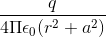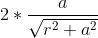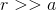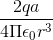Question

(a) Which physical quantity has its SI unit (1) Cm (2) N/C.(b) Two point charges q and -q is placed at a distance $$2a$$ apart. Calculate the electric field at a point P is situated at a distance r along the perpendicular bisector of the line joining the charges. What is the electric field when r >> a? Also, give the direction of electric field w.r.t. electric dipole moment?

Solution

a) 1) Electric dipole moment    2) Electric fieldb) Field at P due to charge q:along BP.Field at P due to charge -q:along PA.Clearly, the components of these 2 forces with equal magnitudes cancel along the direction OP but add up along the direction parallel to BA.Therefore, the resultant electric field at P due to the 2 charges at A and B is:E = -*WhenE = -the direction of electric field in this case is opposite to the direction of the dipole moment vector.Physics

Suggest Corrections0Similar questions
View MorePeople also searched for
View More